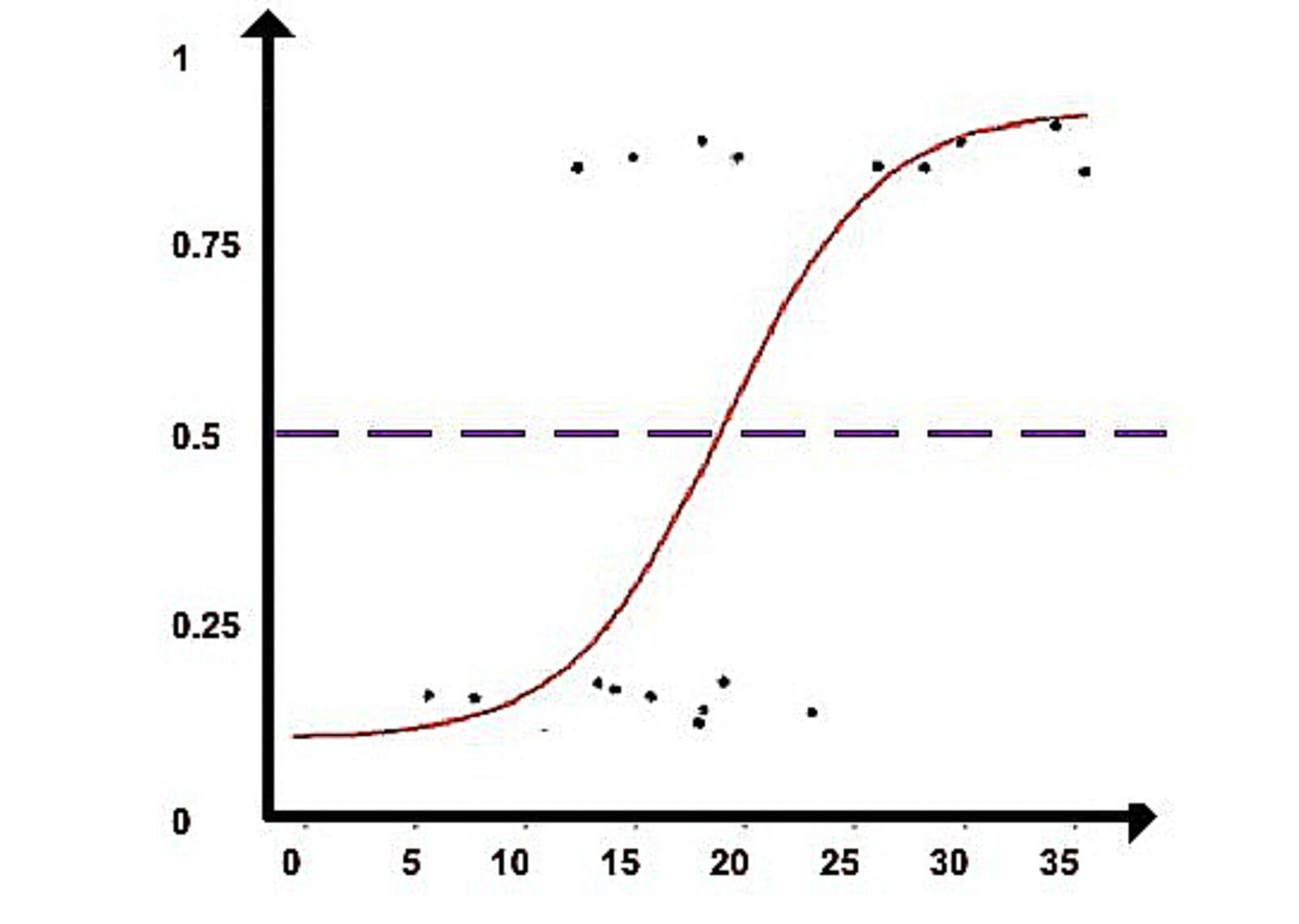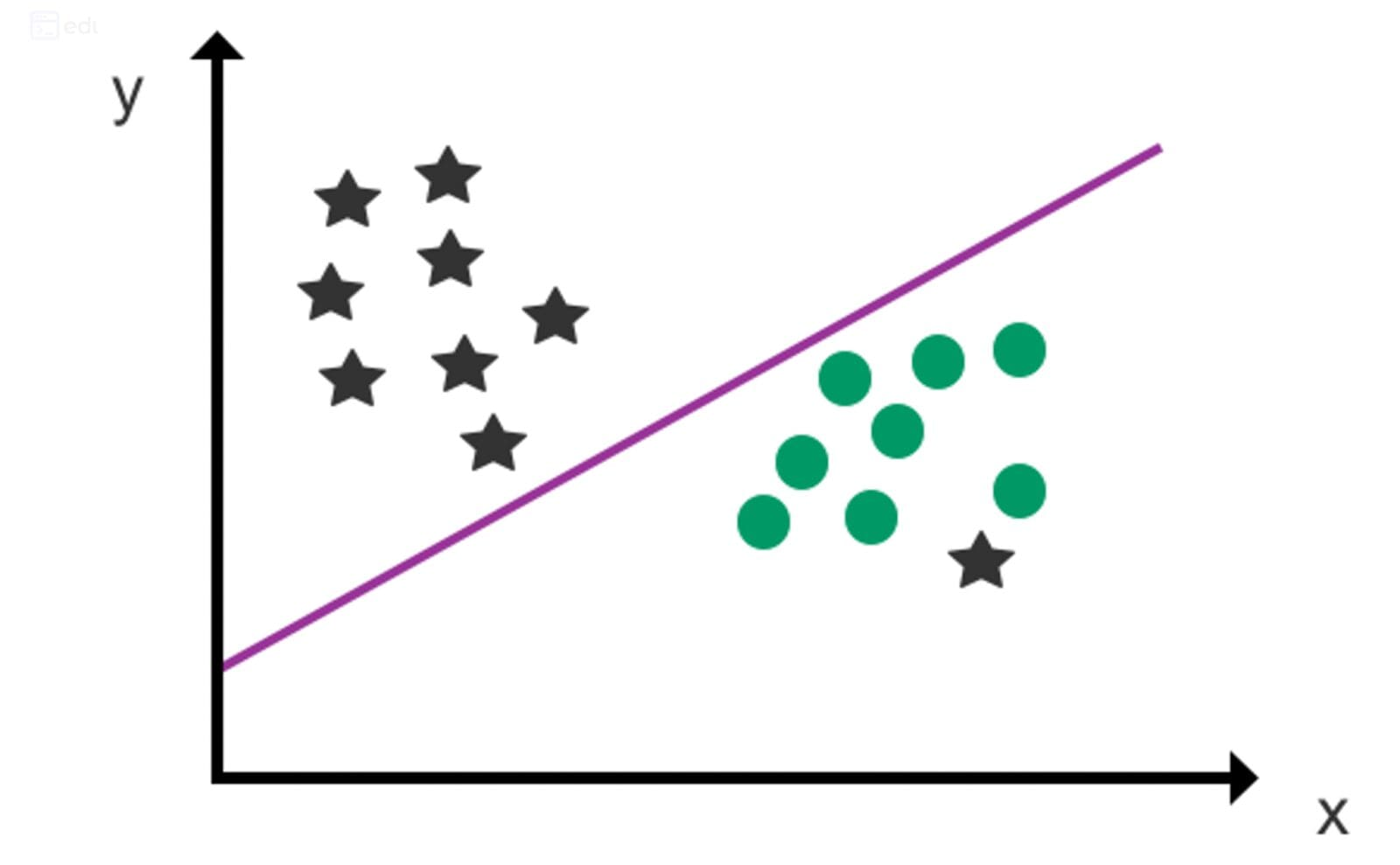# How Does Logistic Regression Work?Image by Editor

In this article, we will be focusing on the Logistic Regression Algorithm. This is a machine learning technique used for classification or prediction in data sets which have many features, but where most of them have little value and should be ignored.

For example, imagine that you have several algorithms for analyzing your photos. Some are good at converting images into text, others are good at detecting objects in photos but not so much for recognizing people’s faces, and others still focus on finding patterns in colour schemes. Now say that you have an image with two people in it: one smiling and one frowning—which one is the happier of the two? Assuming this isn’t something to be kept as a secret (which it probably shouldn’t be), then you need more than just judging by appearances. You want to learn what makes people happy and sad, so instead of having various algorithms that each specialize in identifying different emotions—you want to create a single algorithm that knows how to recognize both emotions equally well. In such cases, using a machine learning algorithm like Logistic Regression can help you achieve your goal more effectively. Learn More.

You can use a multi-class classification algorithm to predict the class of a new data point. For example, an email may be labelled as spam or ham (not spam) for each instance. To create a logistic regression algorithm that works for multi-class and multi-labelling problems, you may add this feature. We will demonstrate how logistic regression works in Scikit Learn for real-time problems in this lecture. This is an example of a binary classification problem. To create a logistic regression algorithm that can work for multi-class and multi-labelling problems, you may add this feature.

Logistic regression is a Machine Learning classification algorithm that is used to predict the probability of certain classes based on some dependent variables. In short, the logistic regression model computes a sum of the input features (in most cases, there is a bias term), and calculates the logistic of the result.

The output of logistic regression is always between (0, and 1), which is suitable for a binary classification task. The higher the value, the higher the probability that the current sample is classified as class=1, and vice versa.

As the formula above shows, is the parameter we want to learn or train or optimize and is the input data. The output is the prediction value when the value is closer to 1, which means the instance is more likely to be a positive sample(y=1). If the value is closer to 0, this means the instance is more likely to be a negative sample(y=0).

To optimize our task, we need to define a loss function(cost or objective function) for this task. In logistic regression, we use the log-likelihood loss function.

m is the number of samples in the training data. is the label of the i-th sample, i is the prediction value of the i-th sample. When the current sample’s label is 1, then the second term of the formula is 0. We hope the larger the first term, the better, and vice versa. Finally, we add the loss of all samples, take the average, and add a negative sign. We want to minimize the quadratic cost function . When is smaller, it means that the model fits better on the data set. There is no closed-form method to find . To achieve this goal, we need to use some optimization algorithms, such as gradient descent. Since is a convex function, the gradient descent is guaranteed to find a global minimum. More Details.

Machine learning generally involves predicting a quantitative outcome or a qualitative class. The former is commonly referred to as a regression problem. In the scenario of linear regression, the input is a continuous variable, and the prediction is a numerical value. When predicting a qualitative outcome (class), the task is considered a classification problem. Examples of classification problems include predicting what products a user will buy or if a target user will click on an online advertisement.

Not all algorithms fit cleanly into this simple dichotomy, though, and logistic regression is a notable example. Logistic regression is part of the regression family as it involves predicting outcomes based on quantitative relationships between variables. However, unlike linear regression, it accepts both continuous and discrete variables as input and its output is qualitative. In addition, it predicts a discrete class such as “Yes/No” or “Customer/Non-customer”.

In practice, the logistic regression algorithm analyzes relationships between variables. It assigns probabilities to discrete outcomes using the Sigmoid function, which converts numerical results into an expression of probability between 0 and 1.0. Probability is either 0 or 1, depending on whether the event happens or not. For binary predictions, you can divide the population into two groups with a cut-off of 0.5. Everything above 0.5 is considered to belong to group A, and everything below is considered to belong to group B.A hyperplane is used as a decision line to separate two categories (as far as possible) after data points have been assigned to a class using the Sigmoid function. The class of future data points can then be predicted using the decision boundary.The logistic regression model is an analysis technique that helps predict the probability of an event happening in the future. This article will explain what logistic regression is, how it works, and how you can use it for forecasting. Logistic regression is a supervised learning method that helps to predict events that have a binary outcome, such as whether a person will successfully pass a driving test. In order to make predictions in this scenario, you need data from past test results. The model takes this data and predicts the likelihood that the same person will pass the test in the future. The main idea behind logistic regression is to use a model based on the probability of an outcome occurring.

Sonia Jessica is a passionate learner and an avid tech blogger.